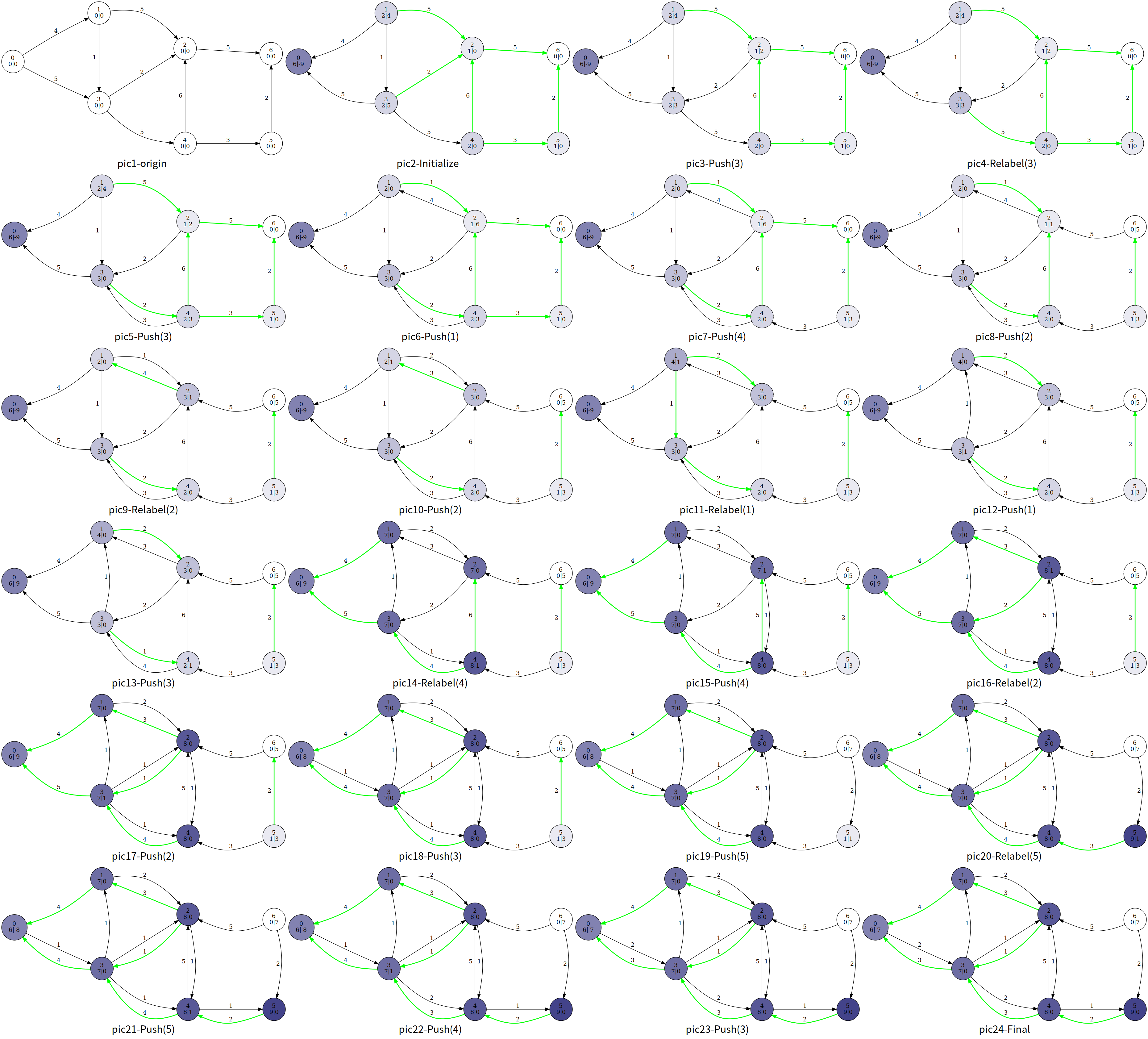# 最大流

## Ford-Fulkerson 增广路算法¶

### 増广路¶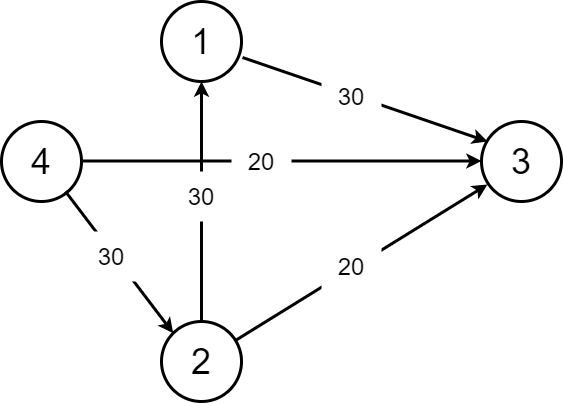1. 这条 增广路 的总流量为 。到 的时候还是 ，到 了就只有 了。

2. 这样子我们就很好的保留了 的流量。

### Edmond-Karp 动能算法（EK 算法）¶

1. 找？我们就从源点一直 BFS 走来走去，碰到汇点就停，然后增广（每一条路都要增广）。我们在 BFS 的时候就注意一下流量合不合法就可以了。

2. 增广？其实就是按照我们找的增广路在重新走一遍。走的时候把这条路的能够成的最大流量减一减，然后给答案加上最小流量就可以了。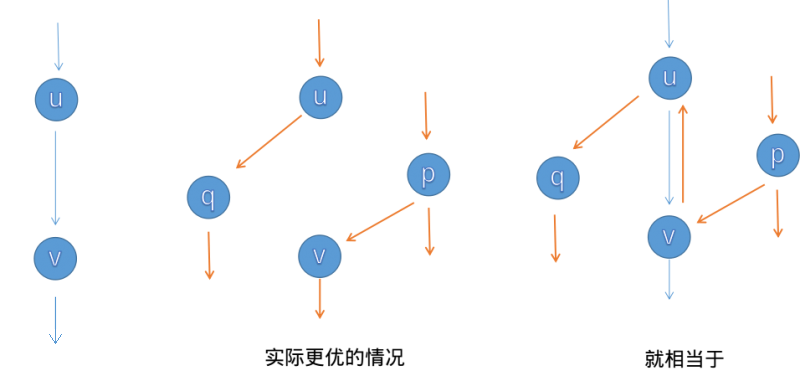EK 算法的时间复杂度为 （其中 为点数， 为边数）。效率还有很大提升空间。

  1 2 3 4 5 6 7 8 9 10 11 12 13 14 15 16 17 18 19 20 21 22 23 24 25 26 27 28 29 30 31 32 33 34 35 36 37 38 39 40 41 42 43 44 45 46 47 48 49 50 51 52 53 54 55 56 57 #define maxn 250 #define INF 0x3f3f3f3f struct Edge { int from, to, cap, flow; Edge(int u, int v, int c, int f) : from(u), to(v), cap(c), flow(f) {} }; struct EK { int n, m; vector edges; vector G[maxn]; int a[maxn], p[maxn]; void init(int n) { for (int i = 0; i < n; i++) G[i].clear(); edges.clear(); } void AddEdge(int from, int to, int cap) { edges.push_back(Edge(from, to, cap, 0)); edges.push_back(Edge(to, from, 0, 0)); m = edges.size(); G[from].push_back(m - 2); G[to].push_back(m - 1); } int Maxflow(int s, int t) { int flow = 0; for (;;) { memset(a, 0, sizeof(a)); queue Q; Q.push(s); a[s] = INF; while (!Q.empty()) { int x = Q.front(); Q.pop(); for (int i = 0; i < G[x].size(); i++) { Edge& e = edges[G[x][i]]; if (!a[e.to] && e.cap > e.flow) { p[e.to] = G[x][i]; a[e.to] = min(a[x], e.cap - e.flow); Q.push(e.to); } } if (a[t]) break; } if (!a[t]) break; for (int u = t; u != s; u = edges[p[u]].from) { edges[p[u]].flow += a[t]; edges[p[u] ^ 1].flow -= a[t]; } flow += a[t]; } return flow; } }; 

### Dinic 算法¶

Dinic 算法 的过程是这样的：每次增广前，我们先用 BFS 来将图分层。设源点的层数为 ，那么一个点的层数便是它离源点的最近距离。

1. 如果不存在到汇点的增广路（即汇点的层数不存在），我们即可停止增广。
2. 确保我们找到的增广路是最短的。（原因见下文）

Dinic 算法有两个优化：

1. 多路增广 ：每次找到一条增广路的时候，如果残余流量没有用完怎么办呢？我们可以利用残余部分流量，再找出一条增广路。这样就可以在一次 DFS 中找出多条增广路，大大提高了算法的效率。
2. 当前弧优化 ：如果一条边已经被增广过，那么它就没有可能被增广第二次。那么，我们下一次进行增广的时候，就可以不必再走那些已经被增广过的边。

  1 2 3 4 5 6 7 8 9 10 11 12 13 14 15 16 17 18 19 20 21 22 23 24 25 26 27 28 29 30 31 32 33 34 35 36 37 38 39 40 41 42 43 44 45 46 47 48 49 50 51 52 53 54 55 56 57 58 59 60 61 62 63 64 65 66 67 68 69 70 71 72 73 74 75 76 #define maxn 250 #define INF 0x3f3f3f3f struct Edge { int from, to, cap, flow; Edge(int u, int v, int c, int f) : from(u), to(v), cap(c), flow(f) {} }; struct Dinic { int n, m, s, t; vector edges; vector G[maxn]; int d[maxn], cur[maxn]; bool vis[maxn]; void init(int n) { for (int i = 0; i < n; i++) G[i].clear(); edges.clear(); } void AddEdge(int from, int to, int cap) { edges.push_back(Edge(from, to, cap, 0)); edges.push_back(Edge(to, from, 0, 0)); m = edges.size(); G[from].push_back(m - 2); G[to].push_back(m - 1); } bool BFS() { memset(vis, 0, sizeof(vis)); queue Q; Q.push(s); d[s] = 0; vis[s] = 1; while (!Q.empty()) { int x = Q.front(); Q.pop(); for (int i = 0; i < G[x].size(); i++) { Edge& e = edges[G[x][i]]; if (!vis[e.to] && e.cap > e.flow) { vis[e.to] = 1; d[e.to] = d[x] + 1; Q.push(e.to); } } } return vis[t]; } int DFS(int x, int a) { if (x == t || a == 0) return a; int flow = 0, f; for (int& i = cur[x]; i < G[x].size(); i++) { Edge& e = edges[G[x][i]]; if (d[x] + 1 == d[e.to] && (f = DFS(e.to, min(a, e.cap - e.flow))) > 0) { e.flow += f; edges[G[x][i] ^ 1].flow -= f; flow += f; a -= f; if (a == 0) break; } } return flow; } int Maxflow(int s, int t) { this->s = s; this->t = t; int flow = 0; while (BFS()) { memset(cur, 0, sizeof(cur)); flow += DFS(s, INF); } return flow; } }; 

### ISAP¶

  1 2 3 4 5 6 7 8 9 10 11 12 13 14 15 16 17 18 19 20 21 22 23 24 25 26 27 28 29 30 31 32 33 34 35 36 37 38 39 40 41 42 43 44 45 46 47 48 49 50 51 52 53 54 55 56 57 58 59 60 61 62 63 64 65 66 67 68 69 70 71 72 73 74 75 76 77 78 79 80 81 82 83 84 85 86 87 88 89 90 91 92 93 94 95 96 97 98 99 100 101 102 103 104 105 106 107 108 109 110 struct Edge { int from, to, cap, flow; Edge(int u, int v, int c, int f) : from(u), to(v), cap(c), flow(f) {} }; bool operator<(const Edge& a, const Edge& b) { return a.from < b.from || (a.from == b.from && a.to < b.to); } struct ISAP { int n, m, s, t; vector edges; vector G[maxn]; bool vis[maxn]; int d[maxn]; int cur[maxn]; int p[maxn]; int num[maxn]; void AddEdge(int from, int to, int cap) { edges.push_back(Edge(from, to, cap, 0)); edges.push_back(Edge(to, from, 0, 0)); m = edges.size(); G[from].push_back(m - 2); G[to].push_back(m - 1); } bool BFS() { memset(vis, 0, sizeof(vis)); queue Q; Q.push(t); vis[t] = 1; d[t] = 0; while (!Q.empty()) { int x = Q.front(); Q.pop(); for (int i = 0; i < G[x].size(); i++) { Edge& e = edges[G[x][i] ^ 1]; if (!vis[e.from] && e.cap > e.flow) { vis[e.from] = 1; d[e.from] = d[x] + 1; Q.push(e.from); } } } return vis[s]; } void init(int n) { this->n = n; for (int i = 0; i < n; i++) G[i].clear(); edges.clear(); } int Augment() { int x = t, a = INF; while (x != s) { Edge& e = edges[p[x]]; a = min(a, e.cap - e.flow); x = edges[p[x]].from; } x = t; while (x != s) { edges[p[x]].flow += a; edges[p[x] ^ 1].flow -= a; x = edges[p[x]].from; } return a; } int Maxflow(int s, int t) { this->s = s; this->t = t; int flow = 0; BFS(); memset(num, 0, sizeof(num)); for (int i = 0; i < n; i++) num[d[i]]++; int x = s; memset(cur, 0, sizeof(cur)); while (d[s] < n) { if (x == t) { flow += Augment(); x = s; } int ok = 0; for (int i = cur[x]; i < G[x].size(); i++) { Edge& e = edges[G[x][i]]; if (e.cap > e.flow && d[x] == d[e.to] + 1) { ok = 1; p[e.to] = G[x][i]; cur[x] = i; x = e.to; break; } } if (!ok) { int m = n - 1; for (int i = 0; i < G[x].size(); i++) { Edge& e = edges[G[x][i]]; if (e.cap > e.flow) m = min(m, d[e.to]); } if (--num[d[x]] == 0) break; num[d[x] = m + 1]++; cur[x] = 0; if (x != s) x = edges[p[x]].from; } } return flow; } }; 

## Push-Relabel 预流推进算法¶

### 通用的预流推进算法¶

，称结点 溢出

#### 通用算法¶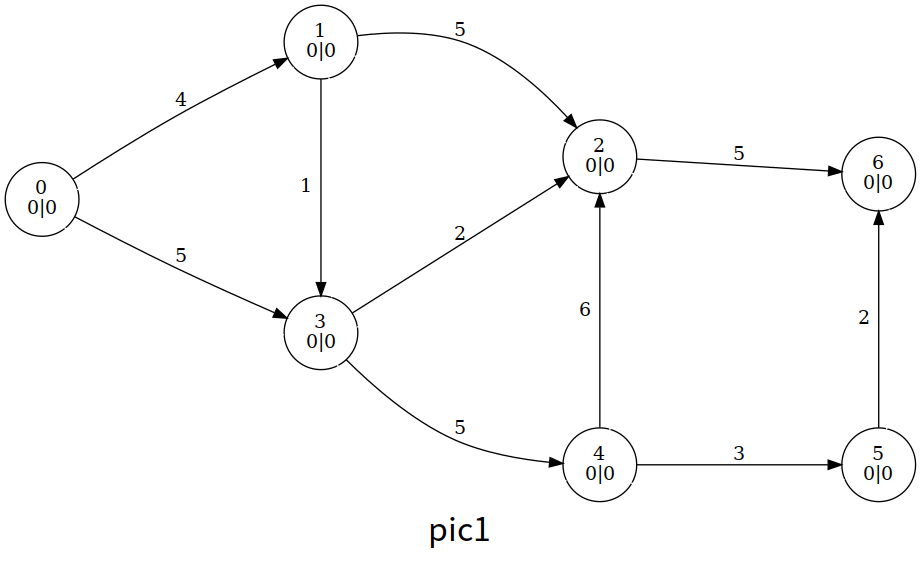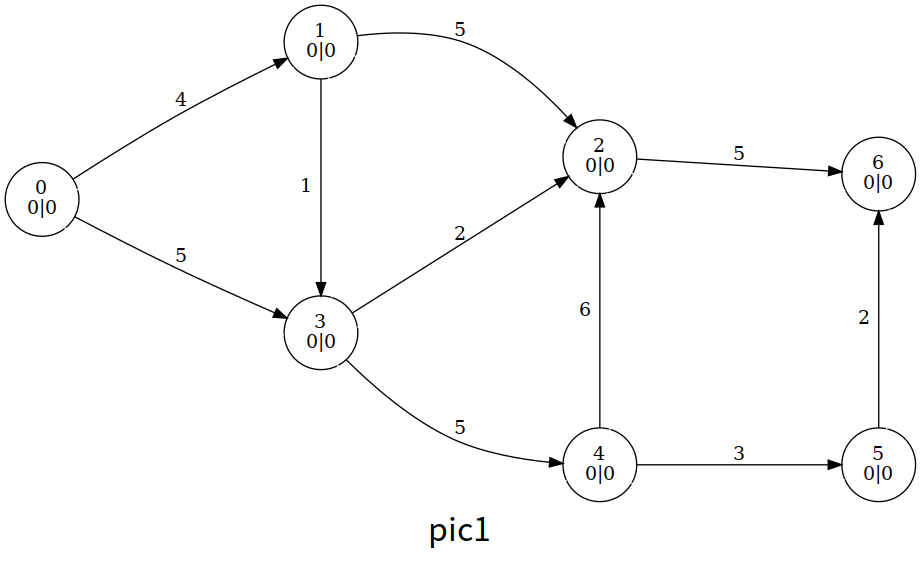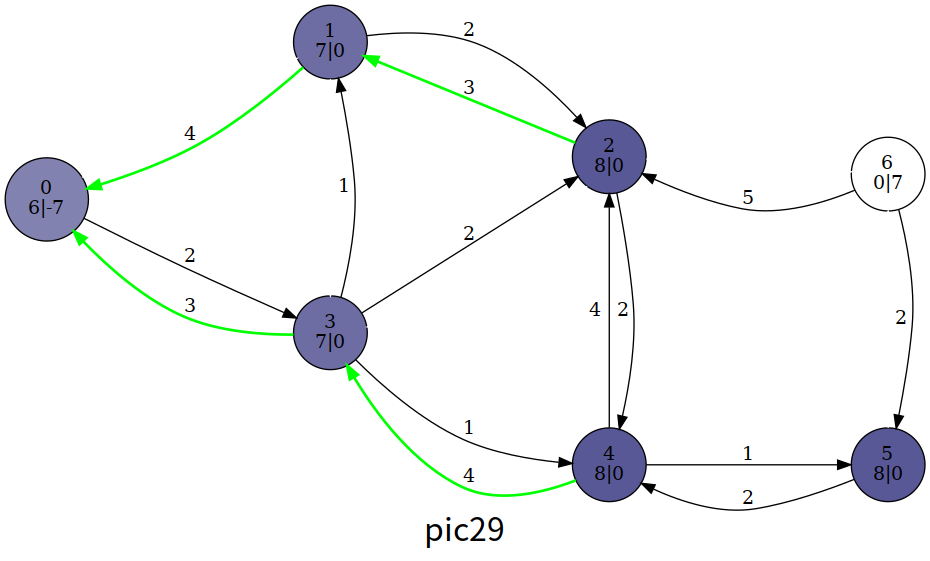1 2 3 4 5 6 7 8 9 10 11 12 13 14 15 16 17 18 19 20 21 22 const int N = 1e4 + 4, M = 1e5 + 5, INF = 0x3f3f3f3f; int n, m, s, t, maxflow, tot; int ht[N], ex[N]; void init() { // 初始化 for (int i = h[s]; i; i = e[i].nex) { const int &v = e[i].t; ex[v] = e[i].v, ex[s] -= ex[v], e[i ^ 1].v = e[i].v, e[i].v = 0; } ht[s] = n; } bool push(int ed) { const int &u = e[ed ^ 1].t, &v = e[ed].t; int flow = min(ex[u], e[ed].v); ex[u] -= flow, ex[v] += flow, e[ed].v -= flow, e[ed ^ 1].v += flow; return ex[u]; // 如果 u 仍溢出，返回 1 } void relabel(int u) { ht[u] = INF; for (int i = h[u]; i; i = e[i].nex) if (e[i].v) ht[u] = min(ht[u], ht[e[i].t]); ++ht[u]; } 

### HLPP 算法¶

1. 初始化（基于预流推进算法）；
2. 选择溢出结点（除 ）中高度最高的结点 ，并对它所有可以推送的边进行推送；
3. 如果 仍溢出，对它重贴标签，回到步骤 2；
4. 如果没有溢出的结点，算法结束。

#### BFS 优化¶

HLPP 的上界为 ，但在使用时卡得比较紧；我们可以在初始化高度的时候进行优化。具体来说，我们初始化 的最短距离；特别地，

#### GAP 优化¶

HLPP 推送的条件是 ，而如果在算法的某一时刻， 的结点个数为 ，那么对于 的结点就永远无法推送超额流到 ，因此只能送回 ，那么我们就在这时直接让他们的高度变成 ，以尽快推送回 ，减少重贴标签的操作。

LuoguP4722【模板】最大流 加强版/预流推进
  1 2 3 4 5 6 7 8 9 10 11 12 13 14 15 16 17 18 19 20 21 22 23 24 25 26 27 28 29 30 31 32 33 34 35 36 37 38 39 40 41 42 43 44 45 46 47 48 49 50 51 52 53 54 55 56 57 58 59 60 61 62 63 64 65 66 67 68 69 70 71 72 73 74 75 76 77 78 79 80 81 82 83 84 85 86 87 88 89 90 91 #include #include #include using namespace std; const int N = 1e4 + 4, M = 2e5 + 5, INF = 0x3f3f3f3f; int n, m, s, t; struct qxx { int nex, t, v; }; qxx e[M * 2]; int h[N], cnt = 1; void add_path(int f, int t, int v) { e[++cnt] = (qxx){h[f], t, v}, h[f] = cnt; } void add_flow(int f, int t, int v) { add_path(f, t, v); add_path(t, f, 0); } int ht[N], ex[N], gap[N]; // 高度；超额流；gap 优化 bool bfs_init() { memset(ht, 0x3f, sizeof(ht)); queue q; q.push(t), ht[t] = 0; while (q.size()) { // 反向 BFS, 遇到没有访问过的结点就入队 int u = q.front(); q.pop(); for (int i = h[u]; i; i = e[i].nex) { const int &v = e[i].t; if (e[i ^ 1].v && ht[v] > ht[u] + 1) ht[v] = ht[u] + 1, q.push(v); } } return ht[s] != INF; // 如果图不连通，返回 0 } struct cmp { bool operator()(int a, int b) const { return ht[a] < ht[b]; } }; // 伪装排序函数 priority_queue, cmp> pq; // 将需要推送的结点以高度高的优先 bool vis[N]; // 是否在优先队列中 int push(int u) { // 尽可能通过能够推送的边推送超额流 for (int i = h[u]; i; i = e[i].nex) { const int &v = e[i].t, &w = e[i].v; if (!w || ht[u] != ht[v] + 1) continue; int k = min(w, ex[u]); // 取到剩余容量和超额流的最小值 ex[u] -= k, ex[v] += k, e[i].v -= k, e[i ^ 1].v += k; // push if (v != s && v != t && !vis[v]) pq.push(v), vis[v] = 1; // 推送之后，v 必然溢出，则入堆，等待被推送 if (!ex[u]) return 0; // 如果已经推送完就返回 } return 1; } void relabel(int u) { // 重贴标签（高度） ht[u] = INF; for (int i = h[u]; i; i = e[i].nex) if (e[i].v) ht[u] = min(ht[u], ht[e[i].t]); ++ht[u]; } int hlpp() { // 返回最大流 if (!bfs_init()) return 0; // 图不连通 ht[s] = n; memset(gap, 0, sizeof(gap)); for (int i = 1; i <= n; i++) if (ht[i] != INF) gap[ht[i]]++; // 初始化 gap for (int i = h[s]; i; i = e[i].nex) { const int v = e[i].t, w = e[i].v; // 队列初始化 if (!w) continue; ex[s] -= w, ex[v] += w, e[i].v -= w, e[i ^ 1].v += w; // 注意取消 w 的引用 if (v != s && v != t && !vis[v]) pq.push(v), vis[v] = 1; // 入队 } while (pq.size()) { int u = pq.top(); pq.pop(), vis[u] = 0; while (push(u)) { // 仍然溢出 // 如果 u 结点原来所在的高度没有结点了，相当于出现断层 if (!--gap[ht[u]]) for (int i = 1; i <= n; i++) if (i != s && i != t && ht[i] > ht[u] && ht[i] < n + 1) ht[i] = n + 1; relabel(u); ++gap[ht[u]]; // 新的高度，更新 gap } } return ex[t]; } int main() { scanf("%d%d%d%d", &n, &m, &s, &t); for (int i = 1, u, v, w; i <= m; i++) { scanf("%d%d%d", &u, &v, &w); add_flow(u, v, w); } printf("%d", hlpp()); return 0; }